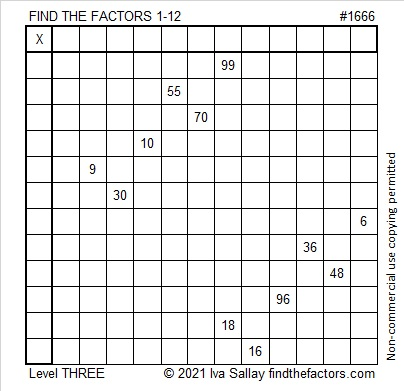# 1666 Demystifying a Tricky Puzzle

Contents

### Today’s Puzzle:

This puzzle isn’t as tricky as it could be simply because I arranged its clues into a level 3 puzzle. That means that after you write the factors of 99 and 18 in the appropriate boxes in the first column and top row, you work your way down the puzzle clue by clue in the order they appear. Still, you will have to think about what to do with the 70, but I think you can handle it!### Factors of 1666:

• 1666 is a composite number.
• Prime factorization: 1666 = 2 × 7 × 7 × 17, which can be written 1666 = 2 × 7² × 17.
• 1666 has at least one exponent greater than 1 in its prime factorization so √1666 can be simplified. Taking the factor pair from the factor pair table below with the largest square number factor, we get √1666 = (√49)(√34) = 7√34.
• The exponents in the prime factorization are 1, 2, and 1. Adding one to each exponent and multiplying we get (1 + 1)(2 + 1)(1 + 1) = 2 × 3 × 2 = 12. Therefore 1666 has exactly 12 factors.
• The factors of 1666 are outlined with their factor pair partners in the graphic below.### More About the Number 1666:

1666 is the sum of two squares because ALL of its odd prime factors either leave a remainder of 1 when divided by 4 OR have an even exponent:
1666 = 2 × 7² × 17,
17÷4 = 4 R1, The exponent on 7² is even.
What are the two squares?
35² +  21² = 1666.

1666 is the hypotenuse of a Pythagorean triple :
784-1470-1666 which is (8-15-17) times 98 and
can also be calculated from 35² –  21², 2(35)(21), 35² +  21².

This site uses Akismet to reduce spam. Learn how your comment data is processed.